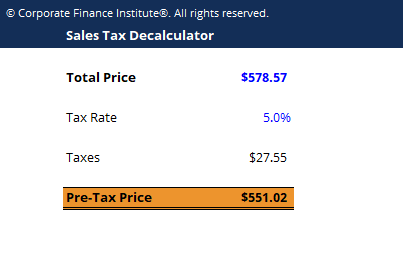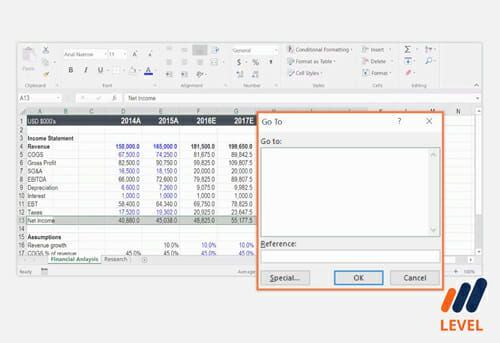# Sales Tax Decalculator

This article gives you a free Sales Tax Decalculator template to use in Excel.

## What is a sales tax decalculator?

A sales tax decalculator will tell you the pre-tax price of a good or service when the total price and tax rate are known.

You can download our free Excel template to determine the pre-tax price.

### How the sales tax decalculator works

The Excel sales tax decalculator works by using a formula that takes the following steps:

Step 1: take the total price and divide it by one plus the tax rate

Step 2: multiply the result from step one by the tax rate to get the dollars of tax

Step 3: subtract the dollars of tax from step 2 from the total price### Download the Free Sales Tax Decalculator Template

Enter your name and email in the form below and download the free template now!

### Sales Tax Decalculator

Download the free Excel template now to advance your finance knowledge!

### Pre-tax price formula used by the Excel decalculator

Here is the formula for decalculating the tax, or determining the pre-tax price of the good/service.

Pre-Tax Price  =  TP – [(TP / (1 + r) x r]

Where:

TP = Total Price

r = Tax rate

### Excel crash course

These types of formulas and functions are covered in detail in our free Excel crash course.### More Excel and finance resources

Thank you for reading CFI’s guide to the Excel sales tax decalculator. If you want to become an Excel power user please check out our Excel Resources to learn all the most important functions, formulas, shortcuts, tips, and tricks.

• Debt schedule
• List of Excel formulas
• Excel templates
• Finance career resources
• Financial modelling courses

### Free Excel Tutorial

To master the art of Excel, check out CFI’s FREE Excel Crash Course, which teaches you how to become an Excel power user.  Learn the most important formulas, functions, and shortcuts to become confident in your financial analysis.

Launch CFI’s Free Excel Course now to take your career to the next level and move up the ladder!